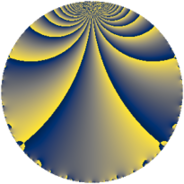Properties

 Label 6160.2.a.bbLevel $6160$ Weight $2$ Character orbit 6160.a Self dual yes Analytic conductor $49.188$ Analytic rank $1$ Dimension $2$ CM no Inner twists $1$

Related objects

Newspace parameters

 Level: $$N$$ $$=$$ $$6160 = 2^{4} \cdot 5 \cdot 7 \cdot 11$$ Weight: $$k$$ $$=$$ $$2$$ Character orbit: $$[\chi]$$ $$=$$ 6160.a (trivial)

Newform invariants

 Self dual: yes Analytic conductor: $$49.1878476451$$ Analytic rank: $$1$$ Dimension: $$2$$ Coefficient field: $$\Q(\sqrt{2})$$ Defining polynomial: $$x^{2} - 2$$ Coefficient ring: $$\Z[a_1, a_2, a_3]$$ Coefficient ring index: $$1$$ Twist minimal: no (minimal twist has level 3080) Fricke sign: $$1$$ Sato-Tate group: $\mathrm{SU}(2)$

$q$-expansion

Coefficients of the $$q$$-expansion are expressed in terms of $$\beta = \sqrt{2}$$. We also show the integral $$q$$-expansion of the trace form.

 $$f(q)$$ $$=$$ $$q + \beta q^{3} + q^{5} - q^{7} - q^{9} +O(q^{10})$$ $$q + \beta q^{3} + q^{5} - q^{7} - q^{9} + q^{11} + ( -2 - \beta ) q^{13} + \beta q^{15} + ( -2 + 3 \beta ) q^{17} -4 \beta q^{19} -\beta q^{21} + 2 q^{23} + q^{25} -4 \beta q^{27} + ( -6 + 2 \beta ) q^{29} + ( 6 - \beta ) q^{31} + \beta q^{33} - q^{35} -2 \beta q^{37} + ( -2 - 2 \beta ) q^{39} + 3 \beta q^{41} + 2 q^{43} - q^{45} + ( 4 + 3 \beta ) q^{47} + q^{49} + ( 6 - 2 \beta ) q^{51} + ( 4 - 6 \beta ) q^{53} + q^{55} -8 q^{57} + ( -6 + \beta ) q^{59} + ( -8 - 3 \beta ) q^{61} + q^{63} + ( -2 - \beta ) q^{65} + ( 2 - 8 \beta ) q^{67} + 2 \beta q^{69} + ( -4 + 6 \beta ) q^{71} + ( -6 - 3 \beta ) q^{73} + \beta q^{75} - q^{77} + ( -10 + 2 \beta ) q^{79} -5 q^{81} + ( -12 - 4 \beta ) q^{83} + ( -2 + 3 \beta ) q^{85} + ( 4 - 6 \beta ) q^{87} + ( -6 + 2 \beta ) q^{89} + ( 2 + \beta ) q^{91} + ( -2 + 6 \beta ) q^{93} -4 \beta q^{95} + ( 2 - 8 \beta ) q^{97} - q^{99} +O(q^{100})$$ $$\operatorname{Tr}(f)(q)$$ $$=$$ $$2 q + 2 q^{5} - 2 q^{7} - 2 q^{9} + O(q^{10})$$ $$2 q + 2 q^{5} - 2 q^{7} - 2 q^{9} + 2 q^{11} - 4 q^{13} - 4 q^{17} + 4 q^{23} + 2 q^{25} - 12 q^{29} + 12 q^{31} - 2 q^{35} - 4 q^{39} + 4 q^{43} - 2 q^{45} + 8 q^{47} + 2 q^{49} + 12 q^{51} + 8 q^{53} + 2 q^{55} - 16 q^{57} - 12 q^{59} - 16 q^{61} + 2 q^{63} - 4 q^{65} + 4 q^{67} - 8 q^{71} - 12 q^{73} - 2 q^{77} - 20 q^{79} - 10 q^{81} - 24 q^{83} - 4 q^{85} + 8 q^{87} - 12 q^{89} + 4 q^{91} - 4 q^{93} + 4 q^{97} - 2 q^{99} + O(q^{100})$$

Embeddings

For each embedding $$\iota_m$$ of the coefficient field, the values $$\iota_m(a_n)$$ are shown below.

For more information on an embedded modular form you can click on its label.

Label $$\iota_m(\nu)$$ $$a_{2}$$ $$a_{3}$$ $$a_{4}$$ $$a_{5}$$ $$a_{6}$$ $$a_{7}$$ $$a_{8}$$ $$a_{9}$$ $$a_{10}$$
1.1
 −1.41421 1.41421
0 −1.41421 0 1.00000 0 −1.00000 0 −1.00000 0
1.2 0 1.41421 0 1.00000 0 −1.00000 0 −1.00000 0
 $$n$$: e.g. 2-40 or 990-1000 Significant digits: Format: Complex embeddings Normalized embeddings Satake parameters Satake angles

Atkin-Lehner signs

$$p$$ Sign
$$2$$ $$1$$
$$5$$ $$-1$$
$$7$$ $$1$$
$$11$$ $$-1$$

Inner twists

This newform does not admit any (nontrivial) inner twists.

Twists

By twisting character orbit
Char Parity Ord Mult Type Twist Min Dim
1.a even 1 1 trivial 6160.2.a.bb 2
4.b odd 2 1 3080.2.a.i 2

By twisted newform orbit
Twist Min Dim Char Parity Ord Mult Type
3080.2.a.i 2 4.b odd 2 1
6160.2.a.bb 2 1.a even 1 1 trivial

Hecke kernels

This newform subspace can be constructed as the intersection of the kernels of the following linear operators acting on $$S_{2}^{\mathrm{new}}(\Gamma_0(6160))$$:

 $$T_{3}^{2} - 2$$ $$T_{13}^{2} + 4 T_{13} + 2$$ $$T_{17}^{2} + 4 T_{17} - 14$$ $$T_{19}^{2} - 32$$ $$T_{23} - 2$$

Hecke characteristic polynomials

$p$ $F_p(T)$
$2$ $$T^{2}$$
$3$ $$-2 + T^{2}$$
$5$ $$( -1 + T )^{2}$$
$7$ $$( 1 + T )^{2}$$
$11$ $$( -1 + T )^{2}$$
$13$ $$2 + 4 T + T^{2}$$
$17$ $$-14 + 4 T + T^{2}$$
$19$ $$-32 + T^{2}$$
$23$ $$( -2 + T )^{2}$$
$29$ $$28 + 12 T + T^{2}$$
$31$ $$34 - 12 T + T^{2}$$
$37$ $$-8 + T^{2}$$
$41$ $$-18 + T^{2}$$
$43$ $$( -2 + T )^{2}$$
$47$ $$-2 - 8 T + T^{2}$$
$53$ $$-56 - 8 T + T^{2}$$
$59$ $$34 + 12 T + T^{2}$$
$61$ $$46 + 16 T + T^{2}$$
$67$ $$-124 - 4 T + T^{2}$$
$71$ $$-56 + 8 T + T^{2}$$
$73$ $$18 + 12 T + T^{2}$$
$79$ $$92 + 20 T + T^{2}$$
$83$ $$112 + 24 T + T^{2}$$
$89$ $$28 + 12 T + T^{2}$$
$97$ $$-124 - 4 T + T^{2}$$